## Selina Concise Mathematics Class 9 ICSE Solutions Area Theorems [Proof and Use]

APlusTopper.com provides step by step solutions for Selina Concise Mathematics Class 9 ICSE Solutions Chapter 16 Area Theorems [Proof and Use]. You can download the Selina Concise Mathematics ICSE Solutions for Class 9 with Free PDF download option. Selina Publishers Concise Mathematics for Class 9 ICSE Solutions all questions are solved and explained by expert mathematic teachers as per ICSE board guidelines.

Selina ICSE Solutions for Class 9 Maths Chapter 16 Area Theorems [Proof and Use]

Exercise 16(A)

Solution 1: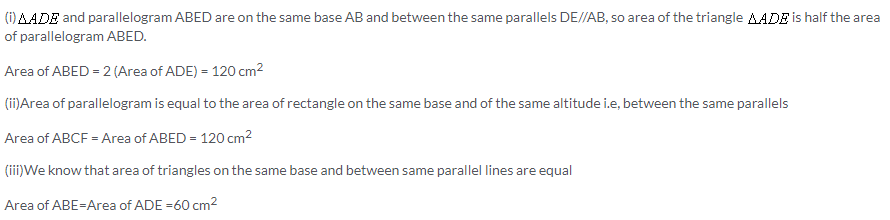Solution 2:Since from the figure, we get CD//FE therefore FC must parallel to DE. Therefore it is proved that the quadrilateral CDEF is a parallelogram.
Area of parallelogram on same base and between same parallel lines is always equal and area of parallelogram is equal to the area of rectangle on the same base and of the same altitude i.e, between same parallel lines.
So Area of CDEF= Area of ABDC + Area of ABEF
Hence Proved

Solution 3: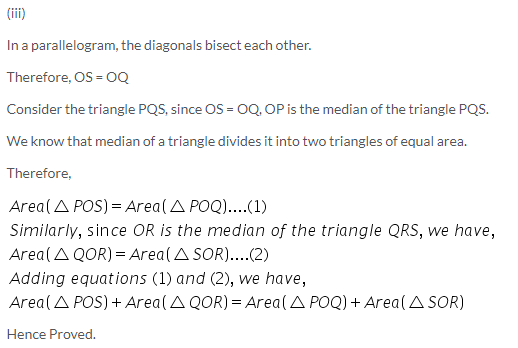Solution 4:Given ABCD is a parallelogram. P and Q are any points on the sides AB and BC respectively, join diagonals AC and BD.
proof:
since triangles with same base and between same set of parallel lines have equal areas
area (CPD)=area(BCD)…… (1)
again, diagonals of the parallelogram bisects area in two equal parts
area (BCD)=(1/2) area of parallelogram ABCD…… (2)
from (1) and (2)
area(CPD)=1/2 area(ABCD)…… (3)
similarly area (AQD)=area(ABD)=1/2 area(ABCD)…… (4)
from (3) and (4)
area(CPD)=area(AQD),
hence proved.
(ii)
We know that area of triangles on the same base and between same parallel lines are equal
So Area of AQD= Area of ACD= Area of PDC = Area of BDC = Area of ABC=Area of APD + Area of BPC
Hence Proved

Solution 5:Solution 6: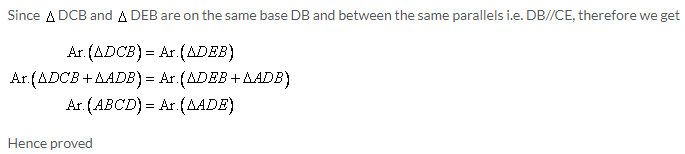Solution 7:Solution 8: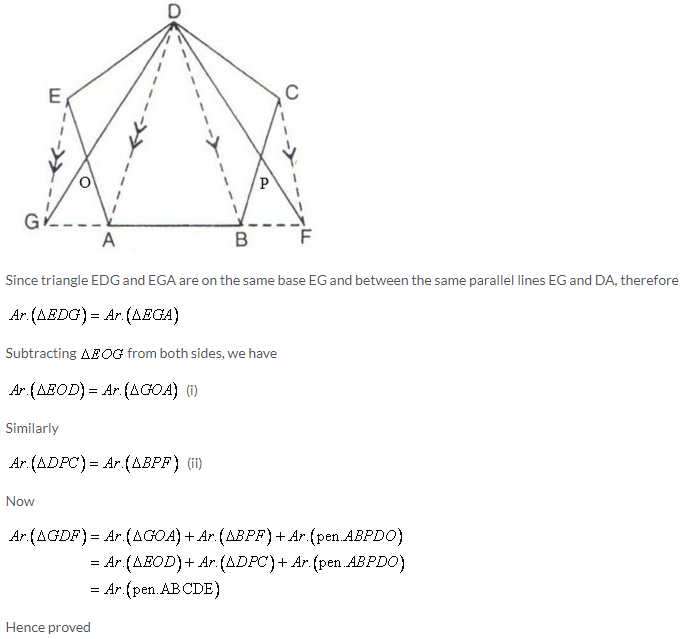Solution 9: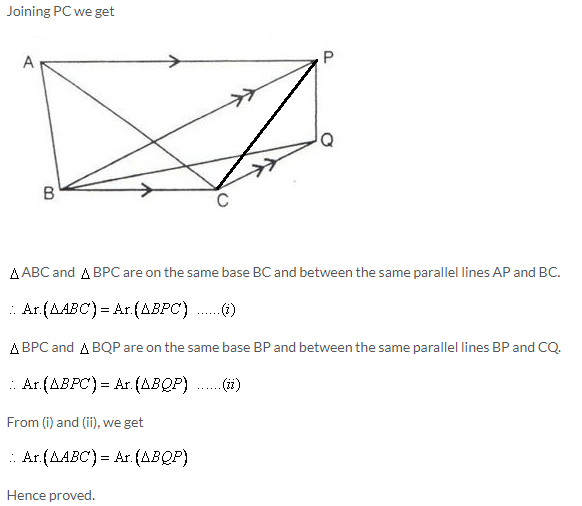Solution 10: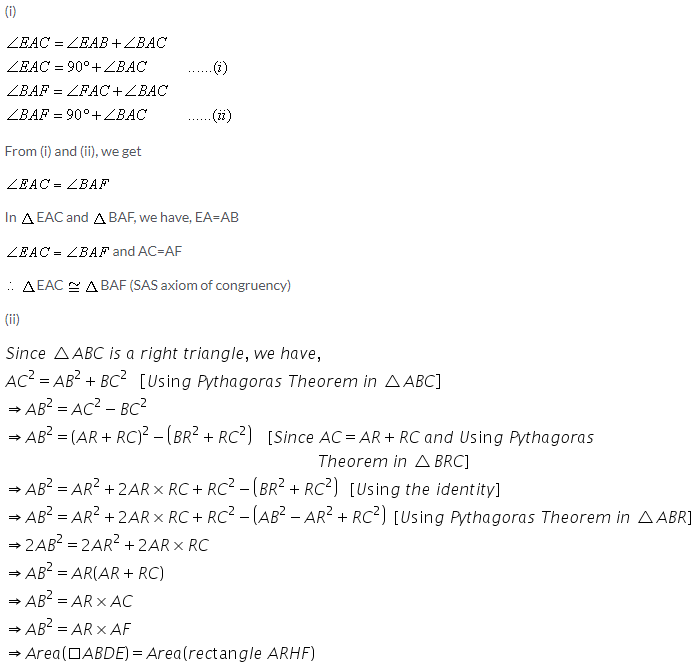Solution 11:Solution 12:Solution 13: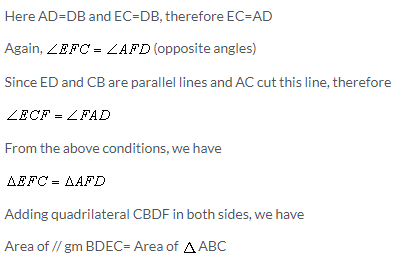Solution 14: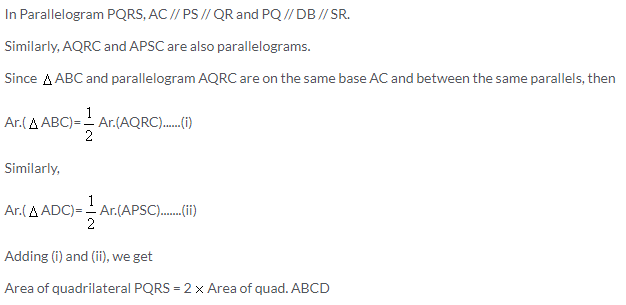Solution 15: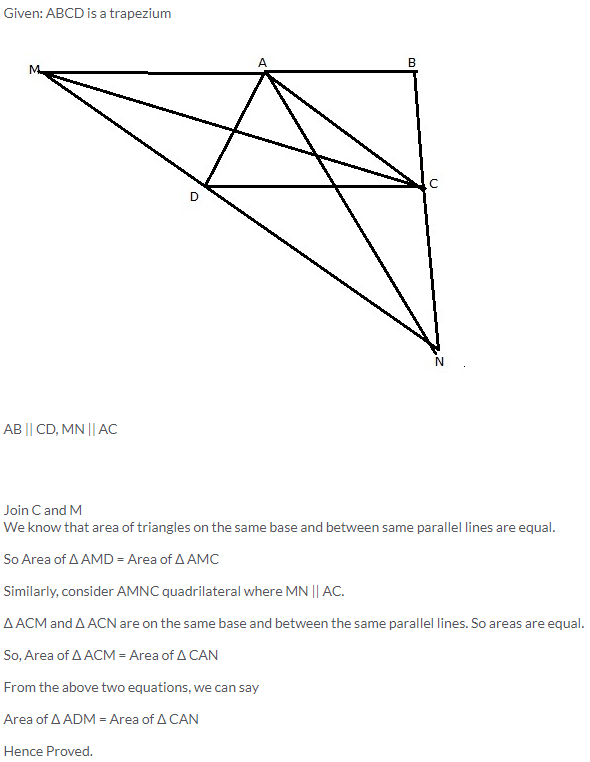Solution 16:
We know that area of triangles on the same base and between same parallel lines are equal.
With common base, BE and between AD and BE parallel lines, we have
Area of ΔABE = Area of ΔBDE
With common base BC and between BE and CF parallel lines, we have
Area of ΔBEC = Area of ΔBEF
Area of ΔABE + Area of ΔBEC = Area of ΔBEF + Area of ΔBDE
=> Area of AEC = Area of DBF
Hence Proved

Solution 17:
Given: ABCD is a parallelogram.
We know that
Area of ΔABC = Area of ΔACD
Consider ΔABX,
Area of ΔABX = Area of ΔABC + Area of ΔACX
We also know that area of triangles on the same base and between same parallel lines are equal.
Area of ΔACX = Area of ΔCXD
From above equations, we can conclude that
Area of ΔABX = Area of ΔABC + Area of ΔACX = Area of ΔACD+ Area of ΔCXD = Area of ACXD Quadrilateral
Hence Proved

Solution 18:
Join B and R and P and R.
We know that the area of the parallelogram is equal to twice the area of the triangle, if the triangle and the parallelogram are on the same base and between the parallels
Consider ABCD parallelogram:
Since the parallelogram ABCD and the triangle ABR lie on AB and between the parallels AB and DC, we haveExercise 16(B)

Solution 1:Solution 2: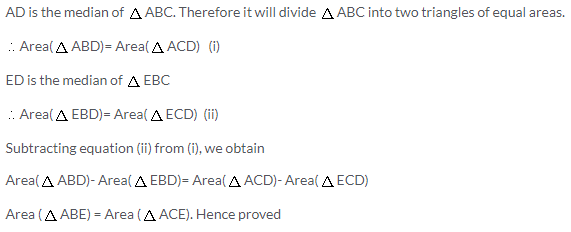Solution 3: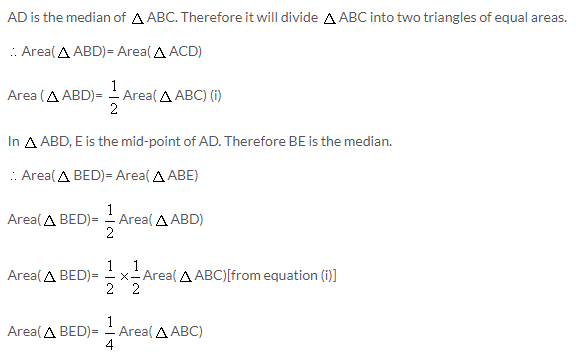Solution 4:
We have to join PD and BD.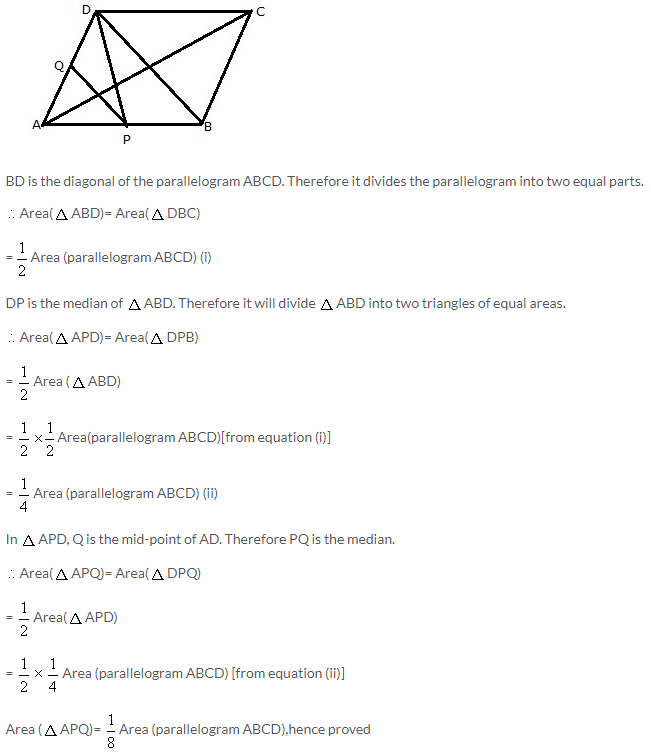Solution 5:Solution 6:
Ratio of area of triangles with same vertex and bases along the same line is equal to ratio of their respective bases. So, we have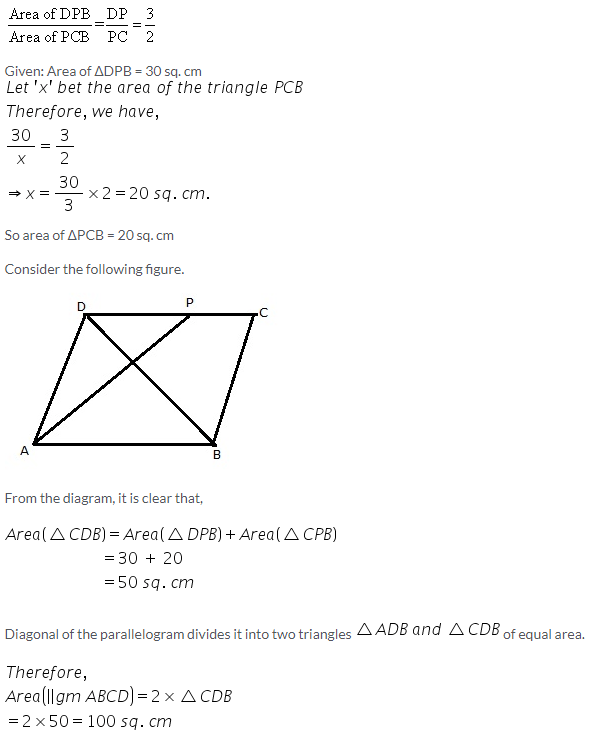Solution 7: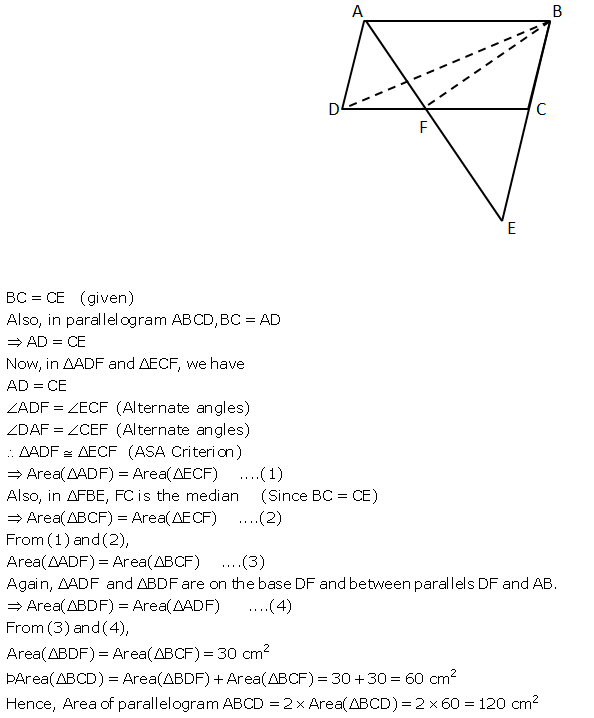Solution 8:Exercise 16(C)

Solution 1: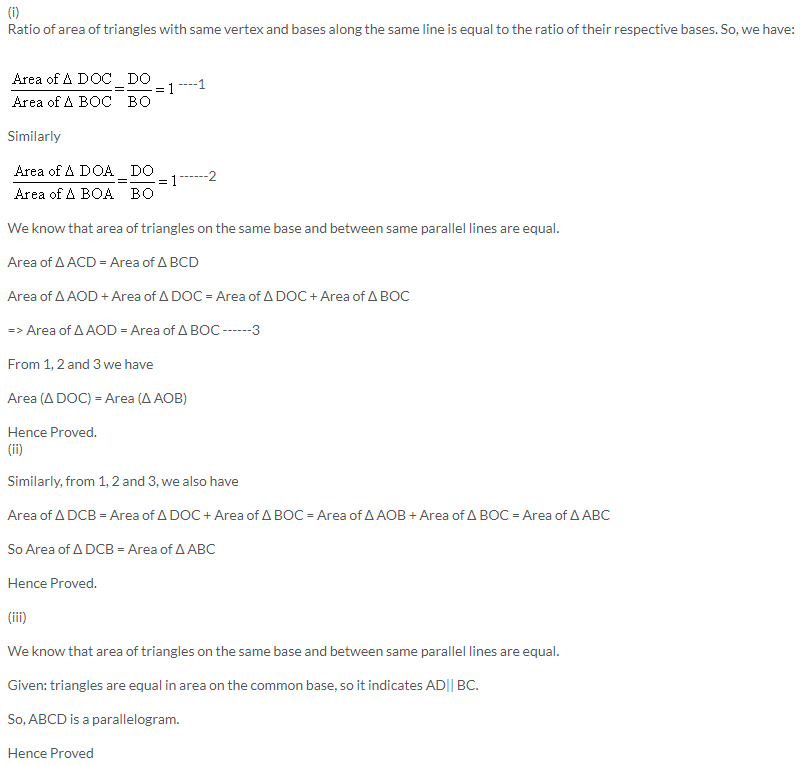Solution 2: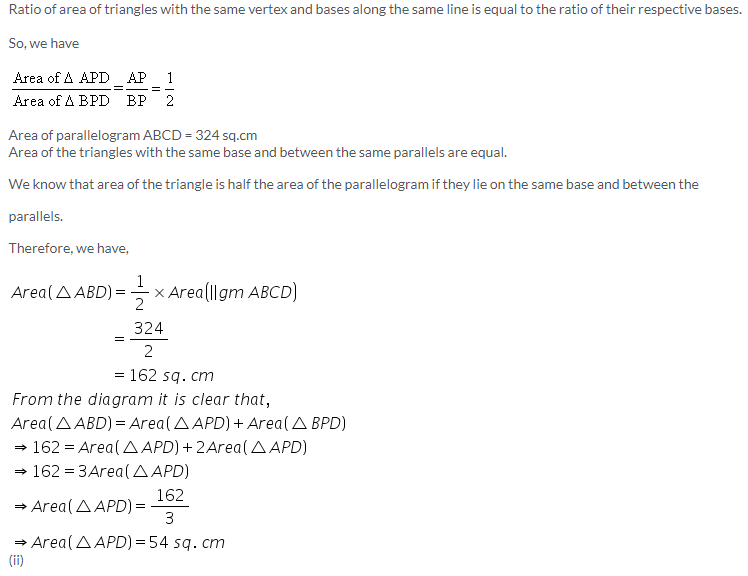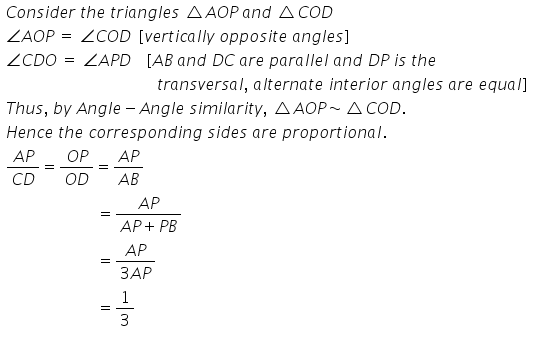Solution 3:Solution 4: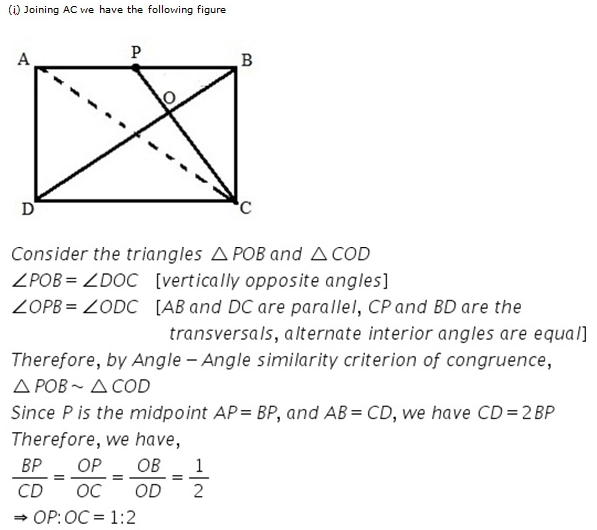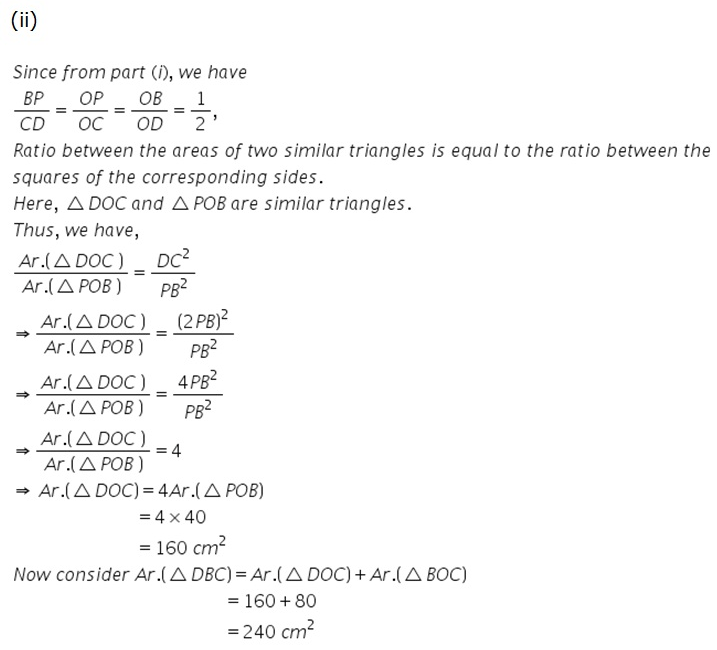Solution 5: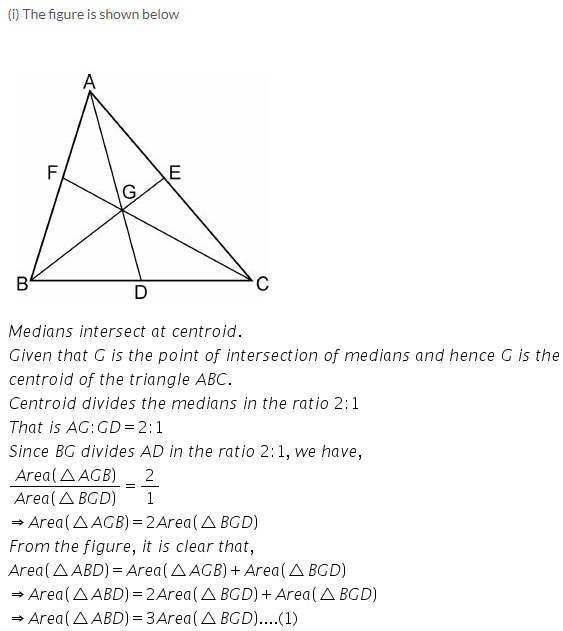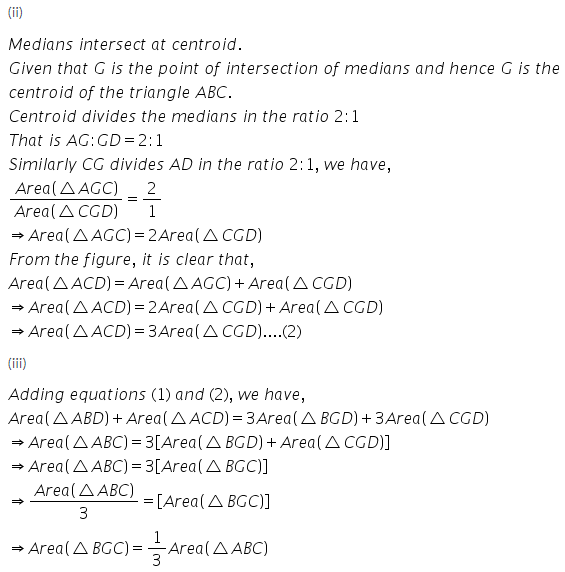Solution 6: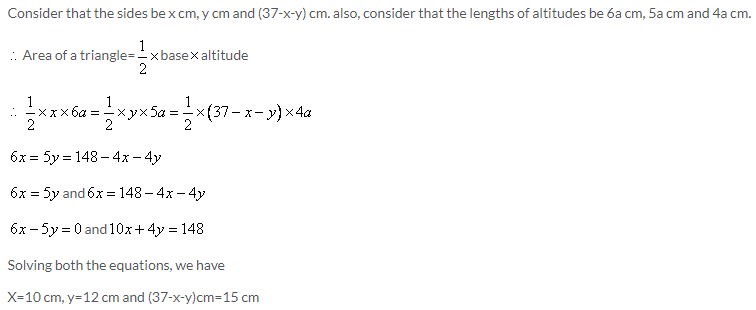Solution 7: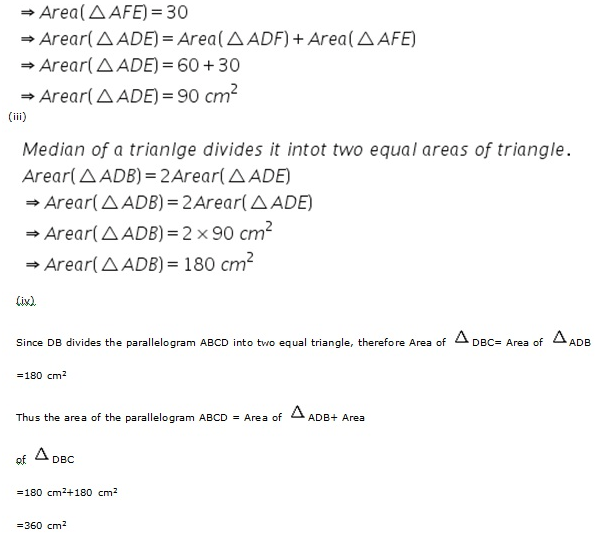Solution 8:Solution 9:More Resources for Selina Concise Class 9 ICSE Solutions